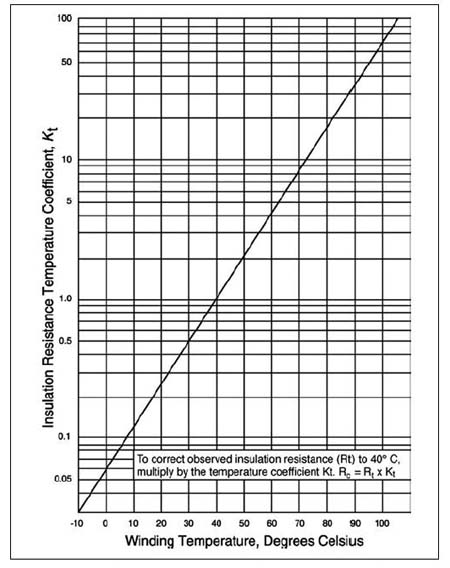# Temperature winding correction

When we perform insulation resistance tester,temperature winding effected the accuracy of Mega Ohms reading.The basic theory is when temperature increase,the insulation resistance decrease due to physical factor of material for winding.

To get a accurate reading for insulation resistance ( Mega Ohms ),we need to ensure the minimum temperature during measurement.

According to IEEE  43,the temperature correction should be to 40 °C and the correction factor for temperature is such that the minimum insulation resistance value is doubled for every 10 °C decrease in winding temperature.

## Temperature winding factor

For example 1 unit induction motor running 24 hour and suddenly it failure.We need to perform insulation resistance measurement to check winding condition,but the temperature of winding around 120 °C.So we must let it cooling down until reach 60 °C to 40 °C before perform insulation resistance test.

Another method if we cannot wait the winding temperature cool until 40 °C,we can use the formula for temperature correction factor.I explain detail below complete with graph and example.

### 1) Graph Insulation resistance versus Temperature## R40 °C = Kt40 °C x Rt

R40 °C = insulation resistance corrected to 40 °C
Rt = measured insulation resistance in MΩ at t °C
Kt40 °C = correction factor according to the graph above

Example for calculation :-

We perform measurement for insulation resistance and get a value 3 MΩ and winding temperature is 70 °C.So the calculation is :

We obtain from the graph : Kt40 °C at 70 °C = 7

Kt40 °C = 7

Rt = 3 MΩ

R40 °C = 7 x  3 MΩ

R40 °C = 21 MΩ

1.Sinnadurai Sripadmanaban says

In the formula given above it appears that the factor (40-T)/10 is not a multiplication factor.
If T is more than 40 it becomes negative

•lemau says

For more easy,i suggest u to use graph Insulation resistance versus temperature to find Kt ( temp. factor ).Sorry for the error.I already replaced new formula.

2.Doug says

Is the graph specific to a certain type of insulation? Regarding transformers, are there different correction factors for oil impregnated paper, silicon impregnated paper, epoxy encapsulated dry types, and varnish dipped dry types?

This site uses Akismet to reduce spam. Learn how your comment data is processed.